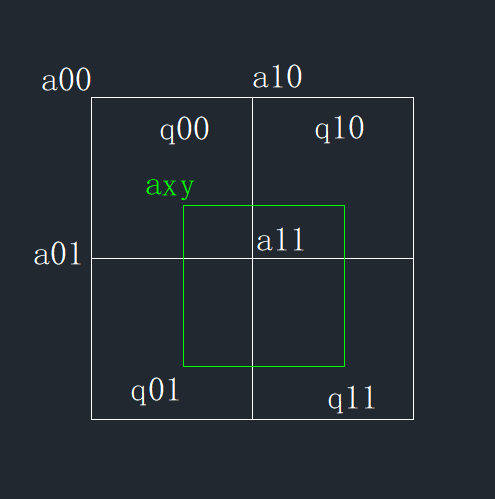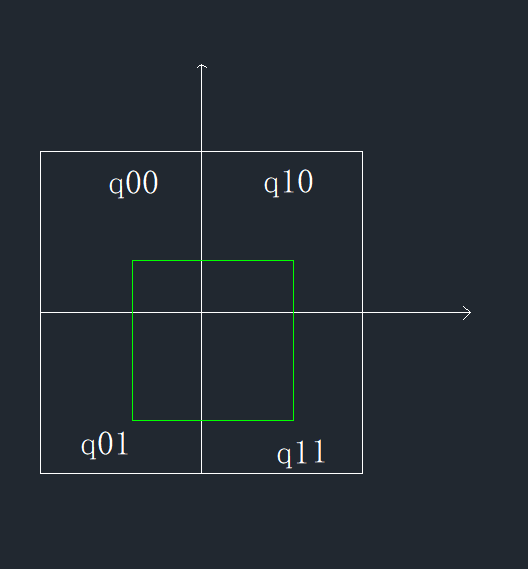# 几何角度理解线性插值和双线性插值${y-y0 \over x-x0} = {y1-y\over x1-x}$

$y = {(x1-x)y0+(x-x0)y1\over x1-x0}$a00,a10,a01,a11是相邻的像素点，其灰度值都已知，求axy的灰度值。$q = (x-x0)(y-y0)q00 + （x+1-x0)(y-y0)q10 + (x-x0)(y+1-y0)q01+(x+1-x0)(y+1-y0)q11$

posted @ 2018-06-15 18:37  Weyne  阅读(...)  评论(...编辑  收藏